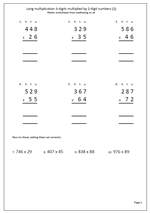# Long multiplication: 3 by 2I am often asked if there are more basic number sheets in the pipeline and so as to not disappoint people here is another page of long multiplication. The first six questions have been laid out in the correct way but the last four have been put in a horizontal position and will need laying out correctly.

The key with multiplying by 2-digit numbers is to multiply by the tens and then the units and finally add the two answers together. Some prefer to multiply by the units first: it makes no difference. Of course when multiplying by the tens digit it is important to remember to place a zero in the units column, ensuring that all other digits are then placed one place to the left and have a value ten times more.

It is also important to keep the numbers in straight columns to make adding up easier.

Long multiplication 3 by 2 (2)